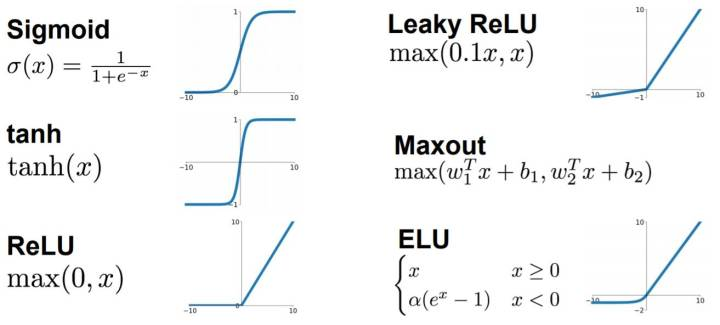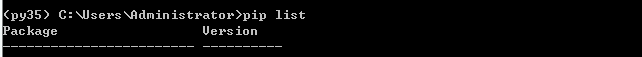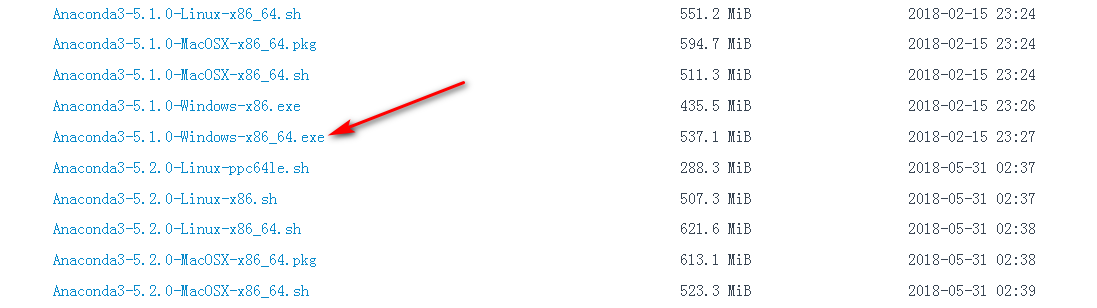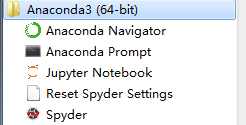2019-03-04 17:25:11 qq_41997920 阅读数 392
• ###### 笔记式Python基础入门

您观看课程学习后 免费入群领取【超全Python资料包+17本学习电子书】 课程简介：以笔记为导向作为讲解内容是本人一贯的授课亮点，一条笔记包含一个知识点，本课程450多条笔记涵盖了python所有入门知识点，非常适合作为入门课程。

2076 人正在学习 去看看 翁老师

## （一）深度学习/神经网络

### 1.神经网络常用的激活函数有哪些？（五种以上）目前最常用的有哪些？

ans：sigmoid函数，tanh函数，ReLU函数 ，Leaky ReLU函数, Maxout函数, ELU函数### 2.为什么要引入激活函数？

ans： 在神经网络中，我们经常可以看到对于某一个隐藏层的节点，该节点的激活值计算一般分为两步：
在进入这个隐藏节点后，会先进行一个线性变换，计算出值 z,再进行一个非线性变换，也就是经过非线性激活函数.

### 3.常用的损失函数有哪些？都用在什么任务上？

ans：损失函数是指用于计算标签和预测值之间的差异

binary_crossentropy,二分类问题，多分类，多标签问题；

categorical_crossentroy,多分类，单标签问题；

mse，回归到任意值；

mse，binary_crossentropy，回归到0-1范围的值；

BCELoss，二分类用的交叉熵

### 4.为什么要设置学习率衰减？常用的学习率衰减方式有哪些？

ans：我们平衡模型的训练速度和损失（loss）后选择了相对合适的学习率（learning rate），但是训练集的损失下降到一定的程度后就不在下降了，遇到这种情况通常可以通过适当降低学习率（learning rate）来实现。但是，降低学习率又会延长训练所需的时间。学习率衰减（learning rate decay）就是一种可以平衡这两者之间矛盾的解决方案。学习率衰减的基本思想是：学习率随着训练的进行逐渐衰减。学习率衰减基本有两种实现方法：

1. 线性衰减。例如：每过5个epochs学习率减半
2. 指数衰减。例如：每过5个epochs将学习率乘以0.1

### 6.什么是分类任务？什么是回归任务？神经网络如何用于这两类任务上？

ans：

• 分类是预测离散类标签输出的问题。
• 回归是预测连续数量输出的问题。

• 回归问题需要预测数量。
• 回归可以具有实值或离散的输入变量。
• 多输入变量的问题通常称为多元回归问题。
• 输入变量按时间排序的回归问题称为时间序列预测问题。

### 7.什么是batch normalization？

ans： 批标准化, 和普通的数据标准化类似, 是将分散的数据统一的一种做法, 也是优化神经网络的一种方法.核心思想是标准化这个过程是可微的，减少不合理的初始化问题，对很坏单独初始化有很强的鲁棒性，同时可以加快网络的手链速度。还可以理解微在网络的每一层前面都会做数据的预处理。

（为什么Batch Normalization那么有用？https://zhuanlan.zhihu.com/p/5274928

（batch normalization 详解 https://blog.csdn.net/gbyy42299/article/details/80363332

### 8.常用的权重初始化方法有哪些？

• Xavier初始化，uniform形式，normal形式；
• MSRA，uniform形式，normal形式；
• Gaussian

## （二）卷积神经网络

### 1.卷积神经网络中的卷积是什么意思?为什么要使用卷积？

ans:参数共享稀疏连接平移不变

• 卷积层只需要很少的参数，就能连接两个很大维度的特征
• 全连接层，要连接两个很大维度的特征，参数会非常多
• 有Pooling层的存在，神经网络具备平移不变这样的性质，提高了泛化能力。

### 2.卷积神经网络与全连接神经网络有什么区别和联系？

ans：相似之处，都是由一些神经元构成，神经元中包含需要学习的参数，通过网络的输入，最后输出结果，通过损失函数优化网络中的参数。

1. 不同之处：网络的层结构不同，全连接神经网络由一系列的隐藏层组成，每个隐藏层由若干个神经元构成，每个神经元与前一层的神经元相关联，但每一层的神经元却是独立的，这样会导致权重的数目激增；卷积神经网络是一个3D容量的神经元，通过宽度，高度，深度排列。

ans:

• 卷积核：“模板窗口”每移动到一个位置，就和图片中的对应元素进行一次卷积运算，注意我们一般把“模板窗口”称为卷积核。特征向量集，提取特征
• 卷积核大小：滤波器的尺寸？3D的权重集合称之为卷积核（参数共享为什么有效是因为：一个特征在不同位置的表现是相同的）
• 卷积步长：卷积步长是指过滤器在图像上滑动的距离；
• feature map：在每个卷积层，数据都是以三维形式存在的。你可以把它看成许多个二维图片叠在一起，其中每一个称为一个feature map；
• pooling：池化层，降低数据体的空间的大小；

### ans:

• Max Pooling Over Time操作
• K-Max Pooling
• Chunk-Max Pooling

（目前只用过Maxpool2d）

ans:

ans:

• LeNet
• AlexNet
• ResNet

### 7.什么叫端到端学习？

ans:从输入端（输入数据）到输出端会得到一个预测结果，与真实结果相比较会得到一个误差，这个误差会在模型中的每一层传递（反向传播），每一层的表示都会根据这个误差来做调整，直到模型收敛或达到预期的效果才结束，这是端到端的。

### 8.用卷积神经网络对图片进行分类和用SVM有什么区别？

ans:神经网络是端到端的训练，不需要人工标注，svm一般需要预先设计和提取特征。

### 1.如何查看pytorch版本？

import torch
print(torch.__version__)  #注意是双下划线### 2.如何查看当前pytorch环境是否支持GPU运算？

torch.cuda.is_available()

### 3.numpy array和pytorch variable如何相互转化?

ans:

1 tensor 转 numpy

a = torch.FloatTensor(3,3)
print a.numpy()

2 numpy 转 tensor

a = np.ones(5)
torch.from_numpy(a)

3 Variable 转 numpy

a = Variable(torch.FloatTensor(3,3))
print a.data.numpy()

4 numpy 转  Variable

a = np.ones(5)
print Variable(torch.from_numpy(a))

ans:

### 5.请解释以下类的初始化参数及用法：

ans：Conv2d：二维卷积 ；

in_channels：输入数据体的深度；

out_channels：输出数据体的深度；

kernel_size：卷积核大小；

stride=1：步长；

dilation=1：表示卷积对输入空间的间隔，默认dilation=1；

groups=1：输入输出数据体深度上的联系，默认groups=1；

bias=True：布尔值，默认bias=True，也表偏执；

ans： MaxPool2d：二维最大池化层；

return_indices=False：表示是否返回最大值所处的下标，默认return_indices=False；

ceil_mode=False：默认ceil_mode=False

(3)  torch.nn.linear(in_features,out_feature,bias=Ture)

ans：torch.nn.Linear类用于定义模型的线性层，即完成前面提到的不同的层之间的线性变换；

in_features：输入特征数；

out_feature：输出特征数。

2019-07-16 03:17:42 kinO_o 阅读数 36
• ###### 笔记式Python基础入门

您观看课程学习后 免费入群领取【超全Python资料包+17本学习电子书】 课程简介：以笔记为导向作为讲解内容是本人一贯的授课亮点，一条笔记包含一个知识点，本课程450多条笔记涵盖了python所有入门知识点，非常适合作为入门课程。

2076 人正在学习 去看看 翁老师

# 深度学习笔记【1】class Neuron: #神经元
def __init__(self, weights, bias):#初始化
self.weights = weights #权重
self.bias = bias  #偏置


Activation函数#sigmoid 函数的函数表达式为：

$H(x)={1\over 1+e^{-x}}$

python 实现如下

def sigmoid(x):
return 1/(1+np.exp(-x))


class Neuron:
def _init_(self,weights,bias):
self.weights=weights
self.bias=bias
def feedforward(self,inputs):
total=np.dot(self,weights,inputs)+self.bias
return sigmoid(total)


Name Weight（Ib） Height Gender
Alice 133 65 F
Bob 160 72 M
Charlie 152 70 M
Diana 120 60 FLoss Function#

$MSE=\frac1n\sum_{i=1}^n (y_{true}-y_{pred})^2$

MSEpython 实现如下

def mse_loss(y_true,y_pred):
return ((y_true-y_pred)**2).mean()


Train#

$\frac{\partial L}{\partial w_i}=\frac{\partial L}{\partial y_{pred}}×\frac{\partial y_{pred}}{\partial h_i}×\frac{\partial h_i}{\partial w_i}$

$w_i\leftarrow w_i - \eta\frac{\partial L}{\partial w_i}$

• 若偏导数是正的，$w_i$要减小，以使$L$减小；
• 若偏导数是负的，$w_i$要增大，以使$L$减小；

import numpy as np
import matplotlib.pyplot as plt
def sigmoid(x):
return 1/(1+np.exp(-x))
def deriv_sigmoid(x):
fx=sigmoid(x)
return fx*(1-fx)
def mse_loss(y_true,y_pred):
return ((y_true-y_pred)**2).mean()
time=np.array(range(0,100))
lost=np.linspace(0,100,100)

class Neuron:
def __init__(self,weights,bias):
self.weights=weights
self.bias=bias

def feedforward(self,inputs):
total=np.dot(self.weights,inputs)+self.bias
return sigmoid(total)

class NeuralNetwork:
def __init__(self):
self.w1=np.random.normal()
self.w2=np.random.normal()
self.w3=np.random.normal()
self.w4=np.random.normal()
self.w5=np.random.normal()
self.w6=np.random.normal()

self.b1=np.random.normal()
self.b2=np.random.normal()
self.b3=np.random.normal()
def feedforward(self,x):
h1=sigmoid(self.w1*x+self.w2*x+self.b1)
h2=sigmoid(self.w3*x+self.w4*x+self.b2)
o1=sigmoid(self.w5*h1+self.w6*h2+self.b3)
return o1
def train(self,data,all_y_trues):
learn_rate=0.1
epochs=1000
for epoch in range(epochs):
for x,y_true in zip(data,all_y_trues):
sum_h1=self.w1*x+self.w2*x+self.b1
h1=sigmoid(sum_h1)
sum_h2=self.w3*x+self.w4*x+self.b2
h2=sigmoid(sum_h2)
sum_o1=self.w5*h1+self.w6*h2+self.b3
o1=sigmoid(sum_o1)
y_pred=o1

#下面计算偏导数，用于改变权值
d_L_d_ypred=-2*(y_true-y_pred)

d_ypred_d_w5=h1*deriv_sigmoid(sum_o1)
d_ypred_d_w6=h2*deriv_sigmoid(sum_o1)
d_ypred_d_b3=deriv_sigmoid(sum_o1)

d_ypred_d_h1 = self.w5 * deriv_sigmoid(sum_o1)
d_ypred_d_h2 = self.w6 * deriv_sigmoid(sum_o1)

d_h1_d_w1 = x * deriv_sigmoid(sum_h1)
d_h1_d_w2 = x * deriv_sigmoid(sum_h1)
d_h1_d_b1 = deriv_sigmoid(sum_h1)

d_h2_d_w3=x*deriv_sigmoid(sum_h2)
d_h2_d_w4=x*deriv_sigmoid(sum_h2)
d_h2_d_b2=deriv_sigmoid(sum_h2)

#更新权值和偏置值
#h1
self.w1-=learn_rate*d_L_d_ypred*d_ypred_d_h1* d_h1_d_w1
self.w2-=learn_rate*d_L_d_ypred*d_ypred_d_h1* d_h1_d_w2
self.b1-=learn_rate*d_L_d_ypred*d_ypred_d_h1* d_h1_d_b1
#h2
self.w3 -= learn_rate * d_L_d_ypred * d_ypred_d_h2 * d_h2_d_w3
self.w4 -= learn_rate * d_L_d_ypred * d_ypred_d_h2 * d_h2_d_w4
self.b2 -= learn_rate * d_L_d_ypred * d_ypred_d_h2 * d_h2_d_b2

# Neuron o1
self.w5 -= learn_rate * d_L_d_ypred * d_ypred_d_w5
self.w6 -= learn_rate * d_L_d_ypred * d_ypred_d_w6
self.b3 -= learn_rate * d_L_d_ypred * d_ypred_d_b3

#计算损失函数
if epoch % 10 == 0:
y_preds = np.apply_along_axis(self.feedforward, 1, data)
loss = mse_loss(all_y_trues, y_preds)
lost[epoch//10] = loss
print("Epoch %d loss: %.3f" % (epoch, loss))

data=np.array([
[-2,-1],
[25,6],
[17,4],
[-15,-6]
])
all_y_trues=np.array([
1,
0,
0,
1,
])
network=NeuralNetwork()
network.train(data,all_y_trues)
plt.plot(time,lost)
plt.show()Vctor Zhou先生的博文

2018-12-26 16:51:43 thu_yang 阅读数 72
• ###### 笔记式Python基础入门

您观看课程学习后 免费入群领取【超全Python资料包+17本学习电子书】 课程简介：以笔记为导向作为讲解内容是本人一贯的授课亮点，一条笔记包含一个知识点，本课程450多条笔记涵盖了python所有入门知识点，非常适合作为入门课程。

2076 人正在学习 去看看 翁老师

# 第一节 Tensorflow运行环境的搭建

1、安装Anaconda

Anaconda 是一个用于科学计算的 Python 发行版，支持 Linux, Mac, Windows, 包含了众多流行的科学计算、数据分析的 Python 包。

Anaconda 安装包可以到 https://mirrors.tuna.tsinghua.edu.cn/anaconda/archive/ 下载。下载完成后，安装即可，安装过程和普通软件安装相同不在叙述。2、安装Tensorflow

conda create -n your_env_name python=3.5

activate your_env_name

pip install tensorflow

2018-05-10 21:36:15 u012950413 阅读数 1812
• ###### 笔记式Python基础入门

您观看课程学习后 免费入群领取【超全Python资料包+17本学习电子书】 课程简介：以笔记为导向作为讲解内容是本人一贯的授课亮点，一条笔记包含一个知识点，本课程450多条笔记涵盖了python所有入门知识点，非常适合作为入门课程。

2076 人正在学习 去看看 翁老师

# 实现一个手写数字识别的算法(使用神经网络算法)

### MNIST数据集:

#神经网络
class Network(object):
def __init__(self, sizes):
self.num_layers = len(sizes)
self.sizes = sizes
self.biases = [np.random.randn(y, 1) for y in sizes[1:]]
self.weights = [np.random.randn(y, x)
for x, y in zip(sizes[:-1], sizes[1:])]

sizes: 每层神经元的个数, 例如: 第一层2个神经元,第二层3个神经元:

                              net = Network([2, 3, 1]);


np.random.rand(y, 1): 随机从正态分布(均值0, 方差1)中生成
net.weights: 存储连接第二层和第三层的权重 (Python索引从0开始数)

${\alpha }^{\prime }=\sigma \left(\omega \ast \alpha +b\right)$

### 正向传播


def feedforward(self, a):
"""Return the output of the network if "a" is input."""
for b, w in zip(self.biases, self.weights): zip函数把两个矩阵（实际上是多个维度不同的向量组成）组合
a = sigmoid(np.dot(w, a)+b)
return a

### 反向传播

随机梯度下降更新公式：


${w}_{k}\to {w}_{k}^{\prime }={w}_{k}-\eta \mathrm{\partial }{C}_{x}/\mathrm{\partial }{w}_{k}$

${b}_{l}\to {b}_{l}^{\prime }={b}_{l}-\eta \mathrm{\partial }{C}_{x}/\mathrm{\partial }{b}_{l}$

#随机梯度下降算法
def SGD(self, training_data list形式, epochs, mini_batch_size 每次用于梯度下降的实例的大小, eta 学习率,
test_data=None):
"""Train the neural network using mini-batch stochastic
gradient descent.  The "training_data" is a list of tuples
"(x 输入特征向量, y x数据所属label)" representing the training inputs and the desired
outputs.  The other non-optional parameters are
self-explanatory.  If "test_data" is provided then the
network will be evaluated against the test data after each
epoch, and partial progress printed out.  This is useful for
tracking progress, but slows things down substantially."""
if test_data: n_test = len(test_data)
n = len(training_data)
for j in xrange(epochs):
random.shuffle(training_data)
mini_batches = [
training_data[k:k+mini_batch_size]
for k in xrange(0, n, mini_batch_size)]
for mini_batch in mini_batches:
self.update_mini_batch(mini_batch, eta)
if test_data:
print "Epoch {0}: {1} / {2}".format(
j, self.evaluate(test_data), n_test)
else:
print "Epoch {0} complete".format(j)

# 反向传播代码
def update_mini_batch(self, mini_batch, eta):
"""Update the network's weights and biases by applying
gradient descent using backpropagation to a single mini batch.
The "mini_batch" is a list of tuples "(x, y)", and "eta"
is the learning rate."""
nabla_b = [np.zeros(b.shape) for b in self.biases]
nabla_w = [np.zeros(w.shape) for w in self.weights]
for x, y in mini_batch:
# 调用backpropagation算法求出偏导数
delta_nabla_b, delta_nabla_w = self.backprop(x, y)
nabla_b = [nb+dnb for nb, dnb in zip(nabla_b, delta_nabla_b)]
nabla_w = [nw+dnw for nw, dnw in zip(nabla_w, delta_nabla_w)]
self.weights = [w-(eta/len(mini_batch))*nw
for w, nw in zip(self.weights, nabla_w)]
self.biases = [b-(eta/len(mini_batch))*nb
for b, nb in zip(self.biases, nabla_b)]
   #这行代码在梯度下降最重要，backpropagation算法下次笔记详细说明
delta_nabla_b, delta_nabla_w = self.backprop(x, y)

### 完整的神经网络实现代码

import random

# Third-party libraries
import numpy as np

class Network(object):

def __init__(self, sizes):
"""The list sizes contains the number of neurons in the
respective layers of the network.  For example, if the list
was [2, 3, 1] then it would be a three-layer network, with the
first layer containing 2 neurons, the second layer 3 neurons,
and the third layer 1 neuron.  The biases and weights for the
network are initialized randomly, using a Gaussian
distribution with mean 0, and variance 1.  Note that the first
layer is assumed to be an input layer, and by convention we
won't set any biases for those neurons, since biases are only
ever used in computing the outputs from later layers."""
self.num_layers = len(sizes)
self.sizes = sizes
self.biases = [np.random.randn(y, 1) for y in sizes[1:]]
self.weights = [np.random.randn(y, x)
for x, y in zip(sizes[:-1], sizes[1:])]

def feedforward(self, a):
"""Return the output of the network if a is input."""
for b, w in zip(self.biases, self.weights):
a = sigmoid(np.dot(w, a)+b)
return a

def SGD(self, training_data, epochs, mini_batch_size, eta,
test_data=None):
"""Train the neural network using mini-batch stochastic
gradient descent.  The training_data is a list of tuples
(x, y) representing the training inputs and the desired
outputs.  The other non-optional parameters are
self-explanatory.  If test_data is provided then the
network will be evaluated against the test data after each
epoch, and partial progress printed out.  This is useful for
tracking progress, but slows things down substantially."""
if test_data: n_test = len(test_data)
n = len(training_data)
for j in xrange(epochs):
random.shuffle(training_data)
mini_batches = [
training_data[k:k+mini_batch_size]
for k in xrange(0, n, mini_batch_size)]
for mini_batch in mini_batches:
self.update_mini_batch(mini_batch, eta)
if test_data:
print "Epoch {0}: {1} / {2}".format(
j, self.evaluate(test_data), n_test)
else:
print "Epoch {0} complete".format(j)

def update_mini_batch(self, mini_batch, eta):
"""Update the network's weights and biases by applying
gradient descent using backpropagation to a single mini batch.
The mini_batch is a list of tuples (x, y), and eta
is the learning rate."""
nabla_b = [np.zeros(b.shape) for b in self.biases]
nabla_w = [np.zeros(w.shape) for w in self.weights]
for x, y in mini_batch:
delta_nabla_b, delta_nabla_w = self.backprop(x, y)
nabla_b = [nb+dnb for nb, dnb in zip(nabla_b, delta_nabla_b)]
nabla_w = [nw+dnw for nw, dnw in zip(nabla_w, delta_nabla_w)]
self.weights = [w-(eta/len(mini_batch))*nw
for w, nw in zip(self.weights, nabla_w)]
self.biases = [b-(eta/len(mini_batch))*nb
for b, nb in zip(self.biases, nabla_b)]

def backprop(self, x, y):
"""Return a tuple (nabla_b, nabla_w) representing the
gradient for the cost function C_x.  nabla_b and
nabla_w are layer-by-layer lists of numpy arrays, similar
to self.biases and self.weights."""
nabla_b = [np.zeros(b.shape) for b in self.biases]
nabla_w = [np.zeros(w.shape) for w in self.weights]
# feedforward
activation = x
activations = [x] # list to store all the activations, layer by layer
zs = [] # list to store all the z vectors, layer by layer
for b, w in zip(self.biases, self.weights):
z = np.dot(w, activation)+b
zs.append(z)
activation = sigmoid(z)
activations.append(activation)
# backward pass
delta = self.cost_derivative(activations[-1], y) * \
sigmoid_prime(zs[-1])
nabla_b[-1] = delta
nabla_w[-1] = np.dot(delta, activations[-2].transpose())
# Note that the variable l in the loop below is used a little
# differently to the notation in Chapter 2 of the book.  Here,
# l = 1 means the last layer of neurons, l = 2 is the
# second-last layer, and so on.  It's a renumbering of the
# scheme in the book, used here to take advantage of the fact
# that Python can use negative indices in lists.
for l in xrange(2, self.num_layers):
z = zs[-l]
sp = sigmoid_prime(z)
delta = np.dot(self.weights[-l+1].transpose(), delta) * sp
nabla_b[-l] = delta
nabla_w[-l] = np.dot(delta, activations[-l-1].transpose())
return (nabla_b, nabla_w)

def evaluate(self, test_data):
"""Return the number of test inputs for which the neural
network outputs the correct result. Note that the neural
network's output is assumed to be the index of whichever
neuron in the final layer has the highest activation."""
test_results = [(np.argmax(self.feedforward(x)), y)
for (x, y) in test_data]
return sum(int(x == y) for (x, y) in test_results)

def cost_derivative(self, output_activations, y):
"""Return the vector of partial derivatives \partial C_x /
\partial a for the output activations."""
return (output_activations-y)

#### Miscellaneous functions
def sigmoid(z):
"""The sigmoid function."""
return 1.0/(1.0+np.exp(-z))

def sigmoid_prime(z):
"""Derivative of the sigmoid function."""
return sigmoid(z)*(1-sigmoid(z))

### 实现一个手写数字识别程序

1.加载mnist数据集
2.查看下返回的训练集、测试集和验证集的数据类型和长度（数据结构搞清楚）
3.创建神经网络
4.进行梯度下降，获得优化后的神经网络模型参数
5.对算法进行准确性评估

#coding=utf-8

from network import Network

# 训练集是一个50000长度的list，每个元素是一个元祖(x,y),x表示输入特征(768),y表示所属数字label
# print(len(trainDataset))
# print(len(trainDataset))
# # x的结构
# print(trainDataset.shape)
# # y的结构
# print(trainDataset.shape)

# list里面是每层神经网络的神经数量
network = Network([784,50,10])
# 进行梯度下降，并测试
network.SGD(trainDataset,30,10,3.0,test_data=testDataset)

2019-03-27 20:06:15 qq_41997920 阅读数 319
• ###### 笔记式Python基础入门

您观看课程学习后 免费入群领取【超全Python资料包+17本学习电子书】 课程简介：以笔记为导向作为讲解内容是本人一贯的授课亮点，一条笔记包含一个知识点，本课程450多条笔记涵盖了python所有入门知识点，非常适合作为入门课程。

2076 人正在学习 去看看 翁老师

## 2.数据读取

import torch
import torchvision
from torchvision import datasets,models,transforms
import os
import matplotlib.pyplot as plt
import time
#torchvision包主要实现数据的处理，导入和预览，以下数据的导入我们将使用这个包

### 2.1数据集处理

1. 训练数据(Training Set)

用于调整网络的权重(weights)和偏差(biases)。

2. 验证数据(Validation Set)

验证数据用于最小化过拟合(overfitting)。训练完成之后，使用测试数据验证其准确度是否满足要求，即验证其推广/泛化能力。

这数据不调整权重和偏差。在基于训练数据调整权重之后，如果基于训练数据的准确度增加了，而基于验证数据的准确度没有增加或反而下降了，则表明过拟合（overfitting）了，需要立即停止训练。

3. 测试数据(Testing Set)

在训练完成之后，使用测试数据确认网络真正的预测和分类能力。

4. Ground Truth

在有监督学习中，数据是有标注的，以(x, t)的形式出现，其中x是输入数据，t是标注。正确的t标注是Ground Truth， 错误的标记则不是。（也有人将所有标注数据都叫做Ground Truth）

### 2.2数据集预览

data_dir = "DogsVSCats"
data_transform = {x:transforms.Compose([transforms.Scale([224,224]),
transforms.ToTensor(),
transforms.Normalize(mean=[0.5,0.5,0.5], std=[0.5,0.5,0.5])])
for x in ["train", "valid"]}

image_datasets = {x:datasets.ImageFolder(root = os.path.join(data_dir,x),
transform = data_transform[x])
for x in ["train", "valid"]}

batch_size = 16,
shuffle = True)
for x in ["train", "valid"]}

Use_gpu = torch.cuda.is_available()

## 3.模型的迁移

model = models.vgg16(pretrained=True)

or parma in model.parameters():
model.classifier = torch.nn.Sequential(torch.nn.Linear(25088, 4096),
torch.nn.ReLU(),
torch.nn.Dropout(p=0.5),
torch.nn.Linear(4096, 4096),
torch.nn.ReLU(),
torch.nn.Dropout(p=0.5),
torch.nn.Linear(4096, 2))
if Use_gpu:
model = model.cuda()

cost = torch.nn.CrossEntropyLoss()
loss_f = torch.nn.CrossEntropyLoss()
optimizer = torch.optim.Adam(model.classifier.parameters(), lr = 0.00001)
epoch_n = 5

time_open = time.time()
for epoch in range(epoch_n):
print("Epoch {}/{}".format(epoch, epoch_n - 1))
print("-"*10)
for phase in ["train", "valid"]:
if phase == "train":
print("Training...")
model.train(True)
else:
print("Validing...")
model.train(False)

running_loss = 0.0
running_corrects = 0
for batch, data in enumerate(dataloader[phase], 1):

X, y = data
if Use_gpu:
X, y = Variable(X.cuda()), Variable(y.cuda())
else:
X, y = Variable(X), Variable(y)

y_pred = model(X)
_, pred = torch.max(y_pred.data, 1)
loss = loss_f(y_pred, y)

if phase == "train":
loss.backward()
optimizer.step()
running_loss += loss.data
running_corrects += torch.sum(pred == y.data)

if batch%500 == 0 and phase =="train":
print("Batch {}, Train Loss:{:.4f}, Train ACC:{:.4f}".format(batch, running_loss/batch, 100*running_corrects(16*batch)))

epoch_loss = running_loss*16/len(image_datasets[phase])
epoch_acc = 100*running_corrects/len(image_datasets[phase])
print("{} Loss:{:.4f} Acc:{:.4f}%".format(phase, epoch_loss,epoch_acc))

time_end = time.time() - time_open
print(time_end)